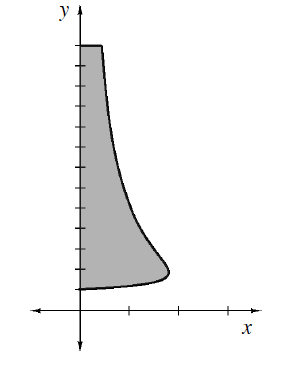### Home > APCALC > Chapter 8 > Lesson 8.1.6 > Problem8-58

8-58.

Suppose the region in problem 8-56 is revolved around the line $x = -1$ instead of the $y$-axis. Set up and evaluate a new integral that will calculate the volume of the solid.Like problem 8-55, the rotating radius will be horizontal (because the axis of rotation is vertical). Consequently, the bounds of integration will be $y$-values: from $y = 1$ to $y = 13$. Both the bounds and the integrand will be written in terms of $y$.

Unlike problem 8-55, there will be a cylindrical hole in the center of this 3D figure. This hole has a radius of $1$.

Unlike problem 8-55, the outer function is no longer $f(y)$. It is $1$ unit longer than $f(y)$.